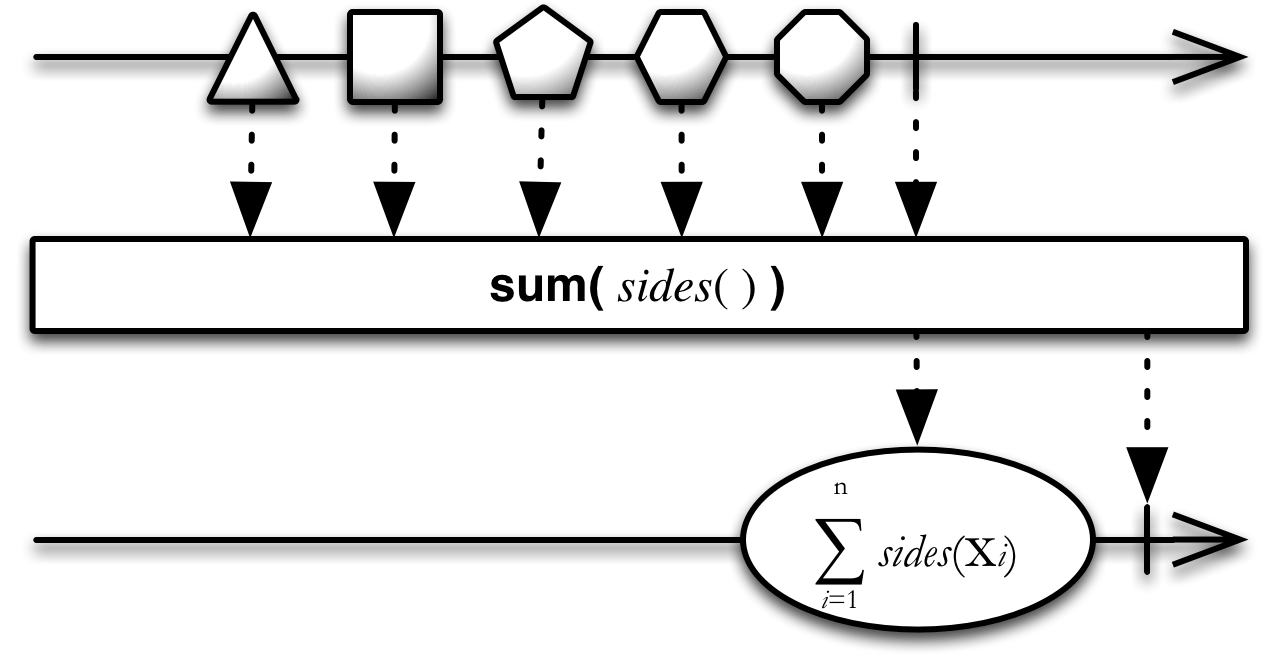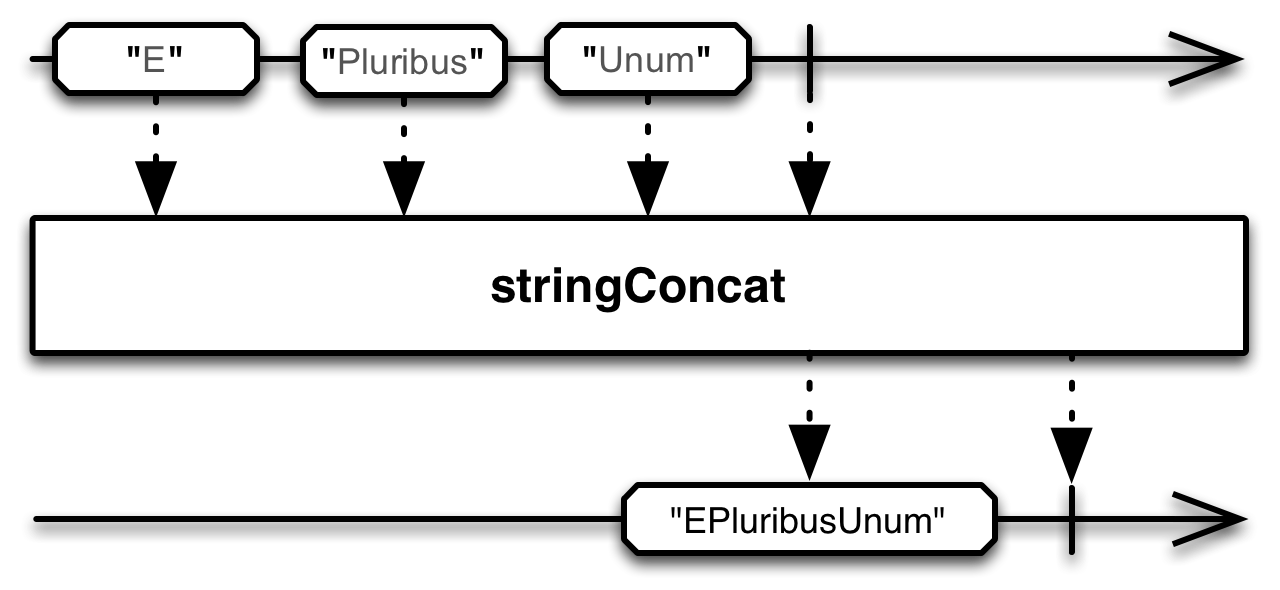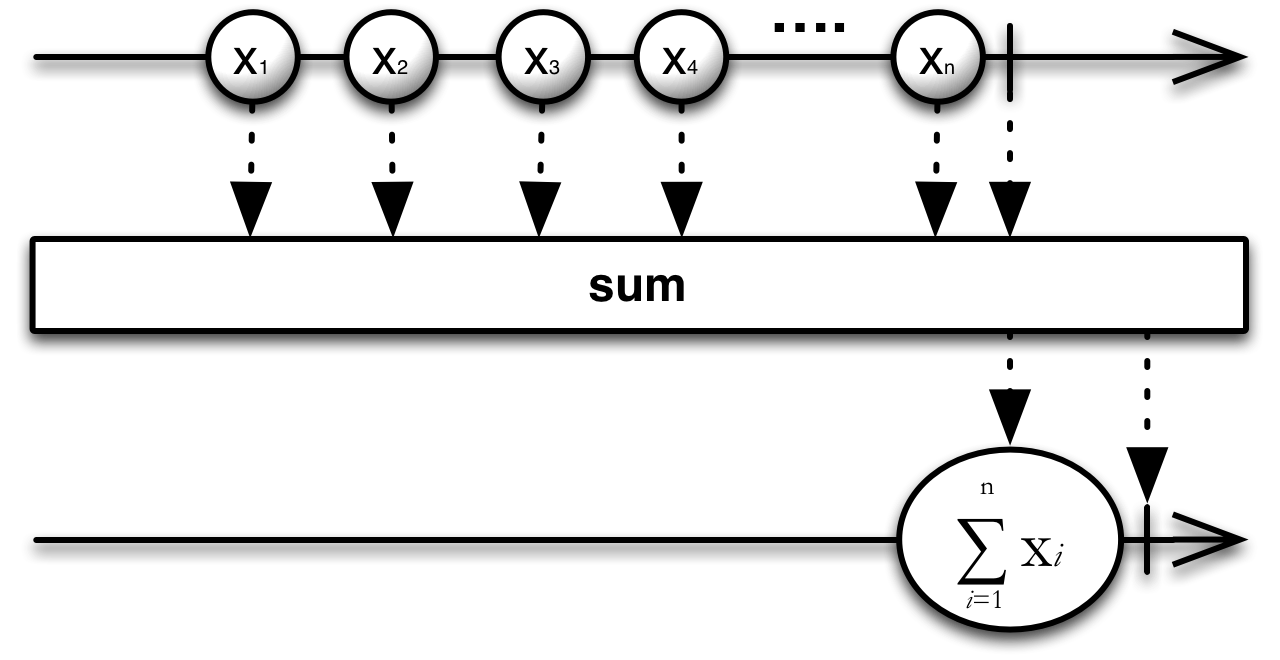/ReactiveX

# Sum

calculates the sum of numbers emitted by an Observable and emits this sum

## Language-Specific Information

In RxGroovy, this operator is not in the ReactiveX core, but is part of the distinct `rxjava-math` module, where it is implemented with four type-specific operators: `sumDouble`, `sumFloat`, `sumInteger`, and `sumLong`. The following example shows how these operators work:

#### Sample Code

```def myObservable = Observable.create({ aSubscriber ->
if(false == aSubscriber.isUnsubscribed()) aSubscriber.onNext(4);
if(false == aSubscriber.isUnsubscribed()) aSubscriber.onNext(3);
if(false == aSubscriber.isUnsubscribed()) aSubscriber.onNext(2);
if(false == aSubscriber.isUnsubscribed()) aSubscriber.onNext(1);
if(false == aSubscriber.isUnsubscribed()) aSubscriber.onCompleted();
});

Observable.sumInteger(myObservable).subscribe(
{ println(it); },                  // onNext
{ println("Error encountered"); }, // onError
{ println("Sequence complete"); }  // onCompleted
);```
```10
Sequence complete```You can also sum not the items themselves but the results of a function applied to each item, as in the illustration above, which emits the sum of the number of sides on the figures emitted by the source Observable.In the distinct StringObservable class (not part of RxGroovy by default) there is also a stringConcat operator that converts an Observable that emits a sequence of strings into an Observable that emits a single string that represents the concatenation of them all.In the distinct StringObservable class (not part of RxGroovy by default) there is also a join operator that converts an Observable that emits a sequence of strings into an Observable that emits a single string that represents the concatenation of each of them, delimited by a string of your choosing.

This operator is not in the RxJava core, but is part of the distinct `rxjava-math` module, where it is implemented with four type-specific operators: `sumDouble`, `sumFloat`, `sumInteger`, and `sumLong`.You can also sum not the items themselves but the results of a function applied to each item, as in the illustration above, which emits the sum of the number of sides on the figures emitted by the source Observable.In the distinct StringObservable class (not part of RxJava by default) there is also a stringConcat operator that converts an Observable that emits a sequence of strings into an Observable that emits a single string that represents the concatenation of them all.In the distinct StringObservable class (not part of RxJava by default) there is also a join operator that converts an Observable that emits a sequence of strings into an Observable that emits a single string that represents the concatenation of each of them, delimited by a string of your choosing.RxJS implements this operator as sum. The following code sample shows how to use it: Sample Code var source = Rx.Observable.range(0, 9).sum(); var subscription = source.subscribe( function (x) { console.log('Next: ' + x); }, function (err) { console.log('Error: ' + err); }, function () { console.log('Completed'); }); Next: 45 CompletedYou can also sum not the items themselves but the results of a function applied to each item, as in the illustration above, which emits the sum number of sides on the figures emitted by the source Observable. Sample Code var arr = [ { value: 1 }, { value: 2 }, { value: 3 } ]; var source = Rx.Observable.fromArray(arr).sum(function (x) { return x.value; }); var subscription = source.subscribe( function (x) { console.log('Next: ' + x); }, function (err) { console.log('Error: ' + err); }, function () { console.log('Completed'); }); Next: 6 Completed

`sum` is found in the following distributions:

• `rx.all.js`
• `rx.all.compat.js`
• `rx.aggregates.js`

It requires one of the following:

• `rx.js`
• `rx.compat.js`
• `rx.lite.js`
• `rx.lite.compat.js`

© ReactiveX contributors
Licensed under the Apache License 2.0.
http://reactivex.io/documentation/operators/sum.html